International
Tables for
Crystallography
Volume D
Physical properties of crystals
Edited by A. Authier

International Tables for Crystallography (2013). Vol. D, ch. 1.11, pp. 270-271

## Section 1.11.2.2.1. Glide-plane forbidden reflections

V. E. Dmitrienko,a* A. Kirfelb and E. N. Ovchinnikovac

aA. V. Shubnikov Institute of Crystallography, Leninsky pr. 59, Moscow 119333, Russia,bSteinmann Institut der Universität Bonn, Poppelsdorfer Schloss, Bonn, D-53115, Germany, and cFaculty of Physics, M. V. Lomonosov Moscow State University, Leninskie Gory, Moscow 119991, Russia
Correspondence e-mail:  dmitrien@crys.ras.ru

#### 1.11.2.2.1. Glide-plane forbidden reflections

| top | pdf |

Considering first the glide-plane forbidden reflections, there may, for instance, exist a glide planeperpendicular to theaxis, i.e. any pointis transformed by this plane into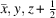. The corresponding matrix of this symmetry operation changes the sign of,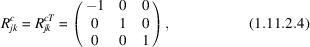and the translation vector into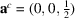. Substituting (1.11.2.4)into (1.11.2.1)and exchanging the integration variables in (1.11.2.3), one obtains for the structure factors of reflections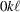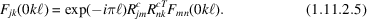If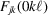is scalar, i.e.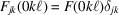, then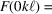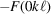for odd, hence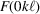vanishes. This is the well known conventional extinction rule for aglide plane, see International Tables for Crystallography Volume A (Hahn, 2005). If, however,is a tensor, the mirror reflection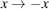changes the signs of theandtensor components [as is also obvious from equation (1.11.2.5)]. As a result, theandcomponents should not vanish for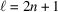and the tensor structure factor becomes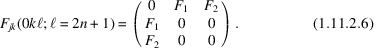In general, the elementsandare complex, and it should be emphasized from the symmetry point of view that they are different and arbitrary for differentand. However, from the physical point of view, they can be readily expressed in terms of tensor atomic factors, where only those chemical elements are relevant whose absorption-edge energies are close to the incident radiation energy (see below).

It is also easy to see that for the non-forbidden (= allowed) reflections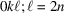, the non-zero tensor elements are just those which vanish for the forbidden reflections: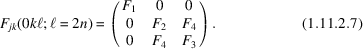Here the result is mainly provided by the diagonal elements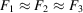, but there is still an anisotropic part that contributes to the structure factor, as expressed by the off-diagonal element. In principle, the effect on the total intensity as well as the element itself can be assessed by careful measurements using polarized radiation.

### References

Hahn, Th. (2005). Editor. International Tables for Crystallography, Volume A, Space-Group Symmetry, 5th ed. Heidelberg: Springer.Google Scholar